Lecture 5: Classical Wave Equations and Solutions

•• Contributed by Delmar Larsen
• Founder and Director at Libretexts

Recap of Lecture 4

Last lecture addressed two important aspects: The Bohr atom and the Heisenberg Uncertainty Principle. The Bohr atom is introduced because is was the first successful description of a quantum atom from basic principles (either as a particle or as a wave, both were discussed). From a particle perspective, stable orbits are predicted from the result of opposing forces (Coloumb's force vs. centripetal force). From a wave perspective, stable "standing waves" are predicted with the wavelength of the electron is a integer times the circumference of the the orbit (otherwise it is not a standing wave and would destructively interfere with itself and disappear). The Bohr atom predicts quantized energies that can be related to Rydberg's phenomenological spectroscopic observation (and decompose his constant $$R$$ into fundamental properties of the universe and matter) via state-to-state transitions (importance for spectroscopy). This is really cool! The problem is that Bohr's theory only applied to hydrogen-like atoms (i..e, atoms or ions with a single electron). So, its quantitative utility for describing quantum chemistry is limited.

Heisenberg's Uncertainly principle is very important and is the realization that trajectories do not exist in quantum mechanics. This is commonly expressed as

$\Delta{p}\Delta{x} \ge \dfrac{h}{4\pi} \nonumber$

and is associated with two properties (in this case, position $$x$$ and momentum $$p$$. As we will show later, not all properties are dictated by Heisenberg's Uncertainly principle. We will also provide a more solid mathematical description of calculating uncertainties (with the standard deviation of a distribution).

Waves Overview

Traveling waves, such as ocean waves or electromagnetic radiation, are waves which “move,” meaning that they have a frequency and are propagated through time and space.A one-dimensional traveling wave at as a function of time. Traveling waves propagate energy from one spot to another with a fixed velocity. Image used with permission from Daniel A. Russell.

In contrast to traveling waves, standing waves, or stationary waves, remain in a constant position with crests and troughs in fixed intervals.A standing wave at two different times (effectively a phase flip with positive being negative and vice versa). Nodes and anti-nodes are pointed out.

Dissecting a Wave

Mathematically, the most basic wave is the (spatially) one-dimensional sine wave (or harmonic wave or sinusoid) with an amplitude $$u$$ described by the equation:

$u(x,t) = A \sin (kx - \omega t + \phi)$

where

• $$A$$ is the maximum amplitude of the wave, maximum distance from the highest point of the disturbance in the medium (the crest) to the equilibrium point during one wave cycle. In the illustration to the right, this is the maximum vertical distance between the baseline and the wave.
• $$x$$ is the space coordinate
• $$t$$ is the time coordinate
• $$k$$ is the wavenumber
• $$\nu$$ is the natural frequency
• $$\omega$$ is the angular frequency (and $$\omega= 2\pi \nu$$)
• $$\phi$$ is the phase (with with respect to what?)The Wave Equation

For a one dimensional wave equation with a fixed length, the function $$u(x,t)$$ describes the position of a string at a specific $$x$$ and $$t$$ value. This leads to the classical wave equation

$\dfrac {\partial^2 u}{\partial x^2} = \dfrac {1}{\nu^2} \cdot \dfrac {\partial ^2 u}{\partial t^2} \label{W1}$

where $$\nu$$ is the velocity of disturbance along the string. Setting boundary conditions as $$x=0$$, $$u(x=0,t) = 0$$ and $$x = \ell$$, $$u(x=\ell , t) = 0$$ allows for this partial differential equation to be solved. Assuming the variables $$x$$ and $$t$$ are independent of each other makes this differential equation easier to solve, as you can separate the variables.

The separation of variables is common method for solving ordinary and partial differential equations, in which algebra allows one to rewrite an equation so that each of two variables occurs on a different side of the equation.

In this case, separation of variables "anzatz" says that

$u(x,t) = X(x)T(t) \label{ansatz}$

Ansatz

"An ansatz is the establishment of the starting equation(s), the theorem(s), or the value(s) describing a mathematical or physical problem or solution. It can take into consideration boundary conditions. After an ansatz has been established (constituting nothing more than an assumption), the equations are solved for the general function of interest (constituting a confirmation of the assumption)." - Wikipedia

Substituting Equation \ref{ansatz} into Equation $$\ref{W1}$$ gives

$T(t) \cdot \dfrac {\partial ^2 X(x)}{\partial x^2} = \dfrac {X(x)}{\nu^2} \cdot \dfrac {\partial ^2 T(t)}{\partial t^2}$

which simplifies to

$\dfrac {1}{X(x)} \cdot \dfrac {\partial ^2 X(x)}{\partial x^2} = \dfrac {1}{T(t)\nu^2} \cdot \dfrac {\partial ^2 T(t)}{\partial t^2} = K$

where $$K$$ is the "separation constant" and $$v$$ is wave velocity. By setting each side equal to $$K$$, two two ordinary differential equations are made.

$\dfrac {d^2 X(x)}{d x^2} - KX(x) = 0$

and

$\dfrac {d^2 T(t)}{d t^2} - K\nu^2 T(t) = 0 \label{time}$

We are particular interest in this example with specific boundary conditions (the wave has zero amplitude at the ends).

$u(x=0,t)=0$

$u(x=L,t)=0$This should sound familiar since we did it for the Bohr hydrogen atom (but with the line curved in on itself)

Solving the Spatial Part

Solving for

$\dfrac {\partial ^2 X(x)}{\partial x^2} - KX(x) = 0 \label{spatial}$

Equation \ref{spatial} is a constant coefficient second order linear ordinary differential equation (ODA), which had general solution of

$X(x) = A\cdot \cos \left(a x \right) + B\cdot \sin \left(b x\right) \label{gen1}$

However, these general solutions can be narrowed down by addressing the boundary conditions. Because of the separation of variables above, $$X(x)$$ has specific boundary conditions (that differ from $$T(t)$$):

• $$X(x=0) = 0$$
• $$X(x=\ell) = 0$$.

So there is not way that any cosine can satisfy the boundary condition (try it if you don't believe me). Hence, $$A=0$$. Moreover, only solution with wavelengths are in integer factors of half the length ($$\ell$$). So Equation \ref{gen1} simplifies to

$X(x) = B\cdot \sin \left(\dfrac {n\pi x}{\ell}\right)$

where $$\ell$$ is the length of the string, $$n = 1, 2, 3, ... \infty$$, and $$B$$ is a constant. By substituting $$X(x)$$ into the partial differential equation, the separation constant is easily obtained to be

$K = -\left(\dfrac {n\pi}{\ell}\right)^2 \label{Kequation}$The first seven $$X(x)$$ solutions of spatial part of the wave equation on a length $$L$$ with two amplitudes (one positive and one negative). Image used with permission from Wikipedia.

Solving the Temporal Part

Plugging the value for $$K$$ from Equation \ref{Kequation} into the temporal component (Equation \ref{time}) and then solving to give the general solution (for the temporal behavior of the wave equation):

$T(t) = D\cos(\omega_n t) + E\sin(\omega_n t) \label{gentime}$

where

• $$D$$ and $$E$$ are constants, and
• $$\omega_n = \dfrac {n\pi\nu}{\ell}$$.

where $$omega$$ is the angular frequency of the oscillation (in time, not space) and $$n$$ is an integer ($$\gt 1$$), which is shared between the spatial and temporal solution.

Unfortunately, we do not have the boundary conditions like with the spatial solution to simplify the expression of the general temporal solutions in Equation \ref{gentime}. However, these solutions can be simplified with basic trigonometry identities to

$T(t) = A_n \cos(\omega_n t +\phi_n)$

where $$A$$ is the maximum displacement of the string (as a function of time), commonly known as amplitude, and $$\phi_n$$ is the phase.

The Total Package: The Spatio-temporal solutions are Standing Waves

Remembering $$u_n (x,t) = X(x) T(t)$$, substituting in our determined $$X$$ and $$T$$ gives

$u_n = A_n \cos(\omega_n t +\phi_n) \sin \left(\dfrac {n\pi x}{\ell}\right)$

The first six wave solutions $$u(x,t;n)$$ are standing waves with frequencies based on the number of nodes (0, 1, 2, 3,...) they exhibit (more discussed in the following Section).The spatio-temporal standing waves solutions to the 1-D wave equation (a string). Image used with permission from Wikipedia.

Since the acceleration of the wave amplitude (right side of Equation 2.1.1) is proportional to $$\dfrac{\partial^2}{\partial x^2}$$, the greater curvature in the material produces a greater acceleration, i.e., greater changing velocity of the wave and greater frequency of oscillation. As discussed later, the higher frequency waves (i..e, more nodes) are higher energy solutions; this as expected from the experiments discussed in Chapter 1 including Plank's equation $$E=h\nu$$.

Superposition

Since the wave equation is a linear homogeneous differential equation, the total solution can be expressed as a sum of all possible solutions.

\begin{align} u(x,t) &= \sum_{n=1}^{\infty} u_n(x,t) \\ &= \sum_{n=1}^{\infty} \left( G_n \cos (\omega_n t) + H_n \sin (\omega_n t) \right) \sin \left(\dfrac{n\pi x}{L}\right) \end{align}

The waveform at a given time is a function of the sources (i.e., external forces, if any, that create or affect the wave) and initial conditions of the system. In many cases (for example, in the classic wave equation), the equation describing the wave is linear. When this is true, the superposition principle can be applied. That means that the net amplitude caused by two or more waves traversing the same space is the sum of the amplitudes which would have been produced by the individual waves separately. For example, two waves traveling towards each other will pass right through each other without any distortion on the other side.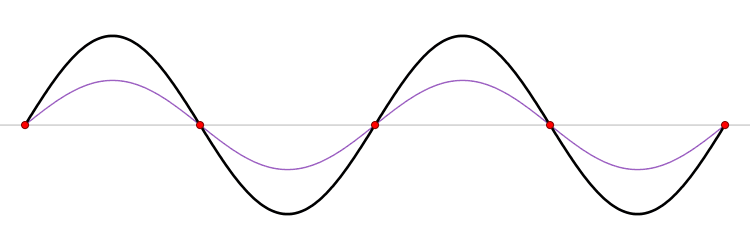Two waves traveling in opposite directions across the same medium combine linearly. In this animation, both waves have the same wavelength and the sum of amplitudes results in a standing wave. Image used with permission (Public Domain; LucasVB)

The $$u_n(x,t)$$ solution is called a normal mode. This sort of expansion is ubiquitous in quantum mechanics.

Superposition in Action

This java applet is a simulation that demonstrates standing waves on a vibrating string. http://www.falstad.com/loadedstring/

Everything above is a classical picture of wave, not specifically quantum, although they all apply. We will introduce quantum tomorrow and the waves will be wavefunctions. Since the Schrödinger equation (that is the quantum wave equation) is linear, the behavior of the original wave function can be computed through the superposition principle.

Expansions is the way of the future

Oscillating Membrane (2D Waves)

Solving for the function $$u(x,y,t)$$ in a vibrating, rectangular membrane is done in a similar fashion by separation of variables, and setting boundary conditions. The solved function is very similar, where

$u(x,y,t) = A_{nm} \cos \left(\omega_{nm} t + \phi_{nm}\right) \sin \left(\dfrac {n_x \pi x}{\ell_a}\right) \sin \left(\dfrac {n_y\pi y}{\ell_b}\right)$

where

• $$\ell_a$$ is the length of the rectangular membrane and $$\ell_b$$ is the width.
• $$n_x$$ and $$n_y$$ are two quantum numbers (one in each dimension)

Now there are now two "quantum numbers" for a 2D standing wave instead of one for the 1D version.

Vibrational Modes of a Rectangular Membrane. Images used with permission from Daniel A. Russell.
The (1,1) Mode The (1,2) Mode The (2,1) Mode The (2,2) Mode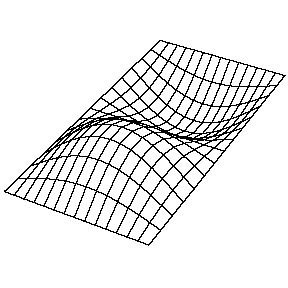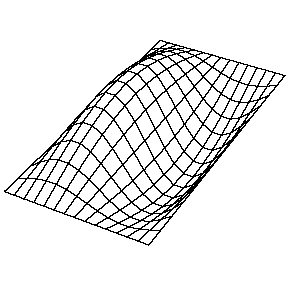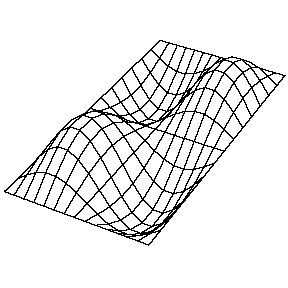Key principle: Degeneracy

These solutions introduces the concept of degeneracy. Two or more different states of a quantum mechanical system are said to be degenerate if they give the same value of energy upon measurement. The number of different states corresponding to a particular energy level is known as the degree of degeneracy of the level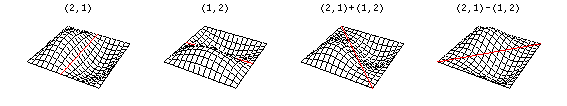The (2,1) and (1,2) normal modes and combinations (all solutions) have the same energy (if the box width and length are identical). Image used with permission from Daniel A. Russell.

If the boundary condition is not a square or rectangle, but a circle, similar solutions can be obtained!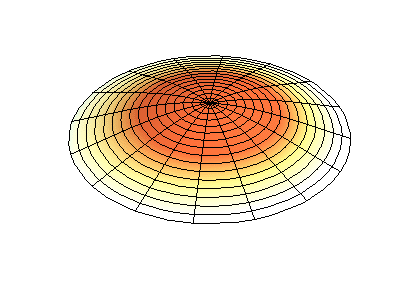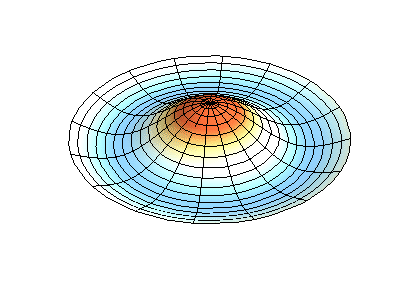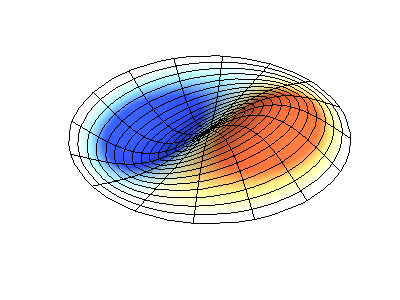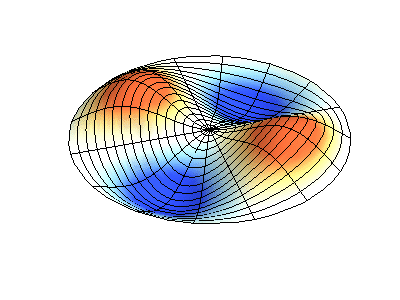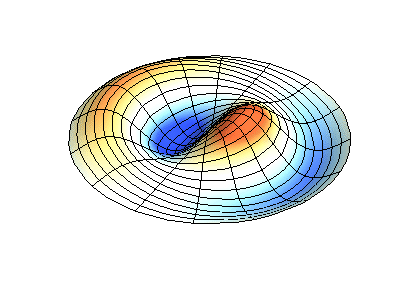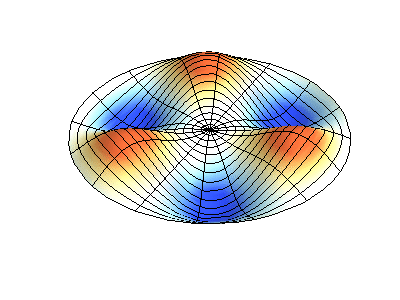Images developed by Dan Russell, Graduate Program in Acoustics, The Pennsylvania State University